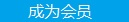您可以捐助，支持我们的公益事业。 1元 10元 50元 认证码：必填求知 文章 文库 Lib 视频 iProcess 课程 认证 咨询 工具 讲座 Modeler Code要资料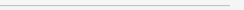订阅捐助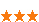287 次浏览     评价： 好 中 差
2019-4-22

 编辑推荐: 本文来自于专知，大家介绍Matplotlib的使用，包括绘图，绘制点和线，以及图像的轮廓和直方图，代码简洁，通俗易懂，旨在让零基础的读者也可以轻松上手！

【导读】在当今互联网蓬勃发展的时代，图像处理技术也随着人们的需求不断进步，专知成员Hui计划推出一系列计算机视觉入门实战讲解，参照Jan Erik Solem编写的《Python计算机视觉编程》这本书，以时下最流行的Python语言为工具，对图像处理技术的具体操作进行详细讲述，上一次的内容中已经为大家介绍了PIL python图像处理类库的使用，包括读取图像，转换灰度图像，创建缩略图，裁剪图像区域，调整尺寸和旋转。Python中有好多工具包应用于图像处理当中，本章作为入门章节，首先来介绍Python中最基本的几个工具包，也希望读者可以在之后自行练习。

Matplotlib

Matplotlib 是一个 Python 的 2D绘图库，它以各种硬拷贝格式和跨平台的交互式环境生成出版质量级别的图形。它可以很好地帮助我们处理数学运算，绘制图表，或者在图像上绘制点、直线和曲线，具有比PIL更强大的绘图功能。Matplotlib中的PyLib接口包含很多方便用户创建图像的函数。

 from PIL import Image from pylab import * x = np.linspace(-np.pi, np.pi, 256, endpoint=True) C, S = np.cos(x), np.sin(x) plt.plot(x, C) plt.plot(x, S) show()

numpy.linspace:在指定的间隔内返回均匀间隔的数字。返回num均匀分布的样本，在[start, stop]。这个区间的端点可以任意的被排除在外。第一个参数为序列的起始点，第二个参数为序列的结束点，第三个参数为步长，第四个参数意为：如果是True，则一定包括stop，如果为False，一定不会有stop。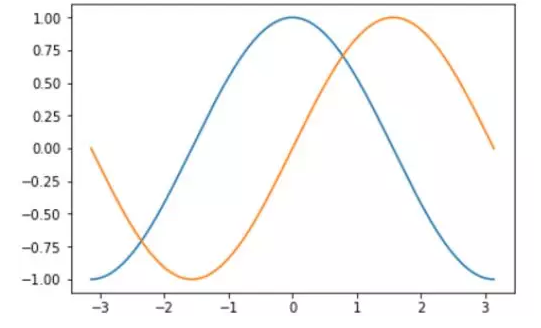plt.plot(x,C,color='red',linewidth=2.5,linestyle='-') plt.plot(x,S,color='blue',linewidth=2.5,linestyle='-.')

 import numpy as np import matplotlib.pyplot as plt x = np.linspace(-np.pi, np.pi, 256, endpoint=True) C, S = np.cos(x), np.sin(x) plt.xlim(x.min() * 1.1, x.max() * 1.1) plt.xticks([-np.pi, -np.pi / 2, 0, np.pi / 2, np.pi], [r'$-\pi$', r'$-\pi/2$', r'$0$', r'$+\pi/2$', r'$+\pi$']) plt.ylim(C.min() * 1.1, C.max() * 1.1) plt.yticks([-1, 0, +1], [r'$-1$', r'$0$', r'$+1$']) plt.plot(x, C, color='red', linewidth=2.5, linestyle='-') plt.plot(x, S, color='blue', linewidth=2.5, linestyle='-.') plt.show()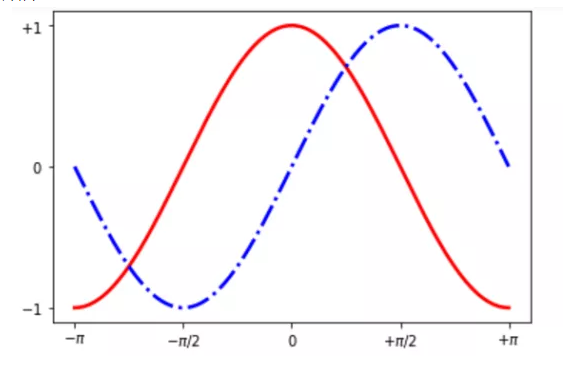Spine(axes, spine_type, path, **kwargs)axes :包含spine的轴实例。spine_type :指定spine类型的字符串path :用于绘制spine的路径实例。例如：我们调用’right’边的spine的’set_color’方法，并且传入’none’参数，这样，左边框的颜色就变成了无色，而’set_position()’方法则使边框的位置改变，“0”代表中心，依靠这个方法我们就能自己“拼凑”出坐标轴，并且能够把其他边框屏蔽掉。’ax.xaxis.set_ticks_position()’方法则设置了坐标轴数据的位置，括号内为传入的位置信息。

 import numpy as np import matplotlib.pyplot as plt ax = plt.subplot(111) ax.spines['right'].set_color('none') ax.spines['top'].set_color('none') ax.xaxis.set_ticks_position('bottom') ax.spines['bottom'].set_position(('data', 0)) ax.yaxis.set_ticks_position('left') ax.spines['left'].set_position(('data', 0)) x = np.linspace(-np.pi, np.pi, 256, endpoint=True) C, S = np.cos(x), np.sin(x) plt.plot(x, C, color='red', linewidth=2.5, linestyle='-', label=r'$cos(t)$') plt.plot(x, S, color='blue', linewidth=2.5, linestyle='-', label=r'$sin(t)$') plt.legend(loc='upper left', frameon=False) plt.xlim(x.min() * 1.1, x.max() * 1.1) plt.xticks([-np.pi, -np.pi / 2, 0, np.pi / 2, np.pi], [r'$-\pi$', r'$-\pi/2$', r'$0$', r'$+\pi/2$', r'$+\pi$']) plt.ylim(C.min() * 1.1, C.max() * 1.1) plt.yticks([-1, 0, +1], [r'$-1$', r'$0$', r'$+1$']) plt.show()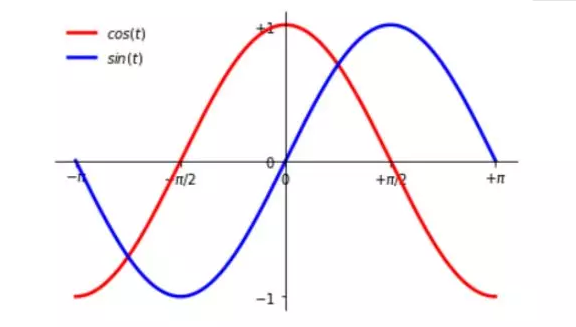from PIL import Image from pylab import * im = array(Image.open("test.jpg")) imshow(im) x = [100, 400, 600, 800] y = [100, 200, 1000, 1200] plot(x[:2], y[:2], 'b*') show()plot(x,y,linewidth=3.5)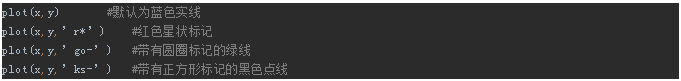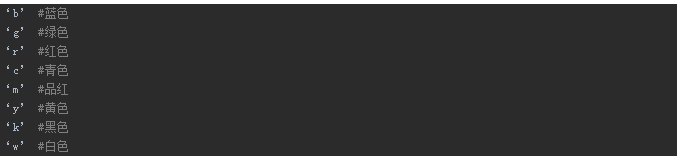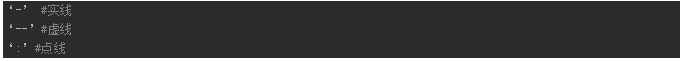from PIL import Image from pylab import * image = array(Image.open('test.jpg').convert('L')) figure() # 新建一个图像 gray() # 不使用颜色信息 contour(image, origin='image') axis('equal') plt.show()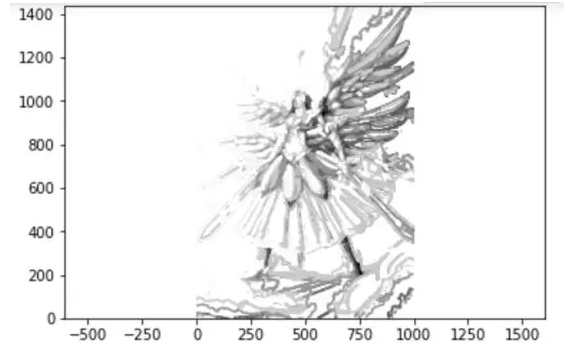contour(Z) ：绘制矩阵Z的等高线

contour(Z,n) ：设置等高线的根数（画出来有n根等高线）

contour(Z,v) ：v是元素随下标单调递增的一维向量，用来设置等高线的值。即在固定的几个值的位置设置等高线。

 from PIL import Image from pylab import * fig = plt.figure(figsize=(15, 15)) image = array(Image.open('test.jpg').convert('L')) plt.subplot(2, 1, 1) plt.imshow(image, cmap=cm.gray) plt.axis("off") plt.subplot(2, 1, 2) plt.hist(image.flatten(), 256) # flatten可以将矩阵转化成一维序列 plt.show()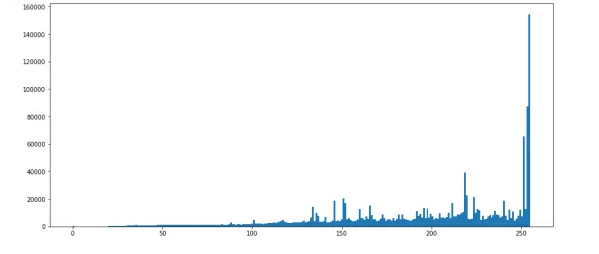hist()函数的第二个参数指定小区间的数目，需要注意的是，因为hist()只接受一维数组作为输入，所以我们在绘制图像直方图之前，必须先对图像进行平压处理，flatten()方法将任意数组按照行优先准则转换成一维数组。

plt.subplot()意为创建子图像。其中括号内传入了三个参数，第一个表示Y轴方向的子图个数，第二个表示X轴方向的子图个数，第三个则表示当前要画图的焦点。287 次浏览  评价: 好 中 差订阅捐助
 相关文章 我们该如何设计数据库 数据库设计经验谈 数据库设计过程 数据库编程总结
 相关文档 数据库性能调优技巧 数据库性能调整 数据库性能优化讲座 数据库系统性能调优系列
 相关课程 高性能数据库设计与优化 高级数据库架构师 数据仓库和数据挖掘技术 Hadoop原理、部署与性能调优每天2个文档/视频 扫描微信二维码订阅
 订阅技术月刊 获得每月300个技术资源